# How to generate random positive numbers without duplicates in Excel?

In this article, the user will learn the method to generate random positive numbers without duplicate values in Excel. This article briefs learners on two strategies to perform this same task. The first example uses VBA code to generate unique random numbers, while the second example will perform the same task by using the kutool. The main point to understand while performing a task is that a single task can be done in many ways. So, learners should know which one should be used to generate the results accurately and precisely.

## Example 1: To generate random number in excel without duplicates by using the predefined formula

### Step 1

Consider the below given Excel sheet, to generate the random number in Excel without generating duplicate values. In this example, VBA code is used to generate random values.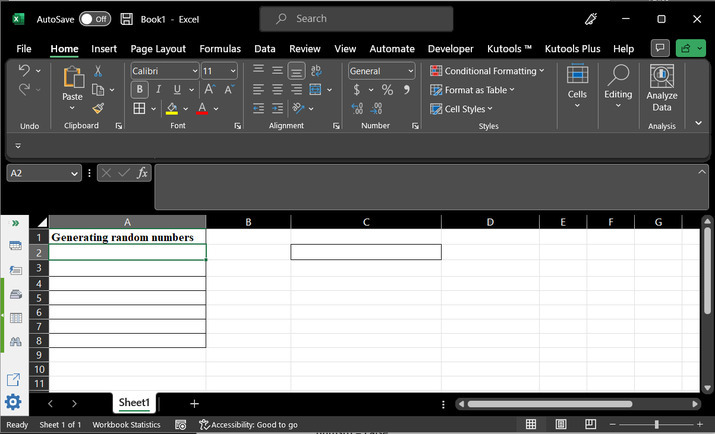### Step 2

Go to the “Developer” tab, and then under the “Code” section select the option “Visual Basic”. This will open a new dialog box.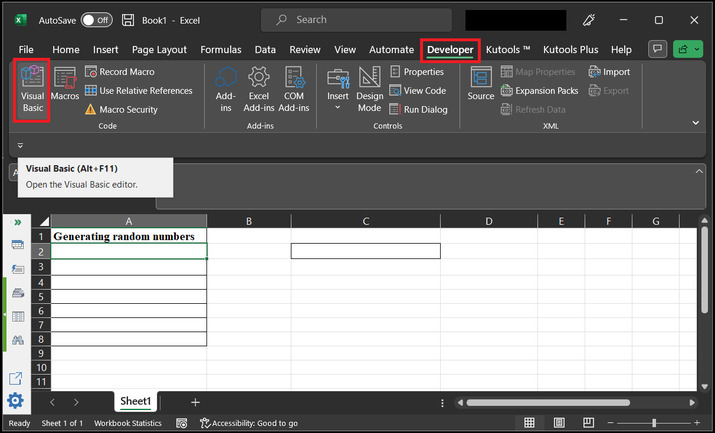### Step 3

The new open dialog box contains the heading “Microsoft Visual Basic for Applications”. Consider the below depicted image for reference −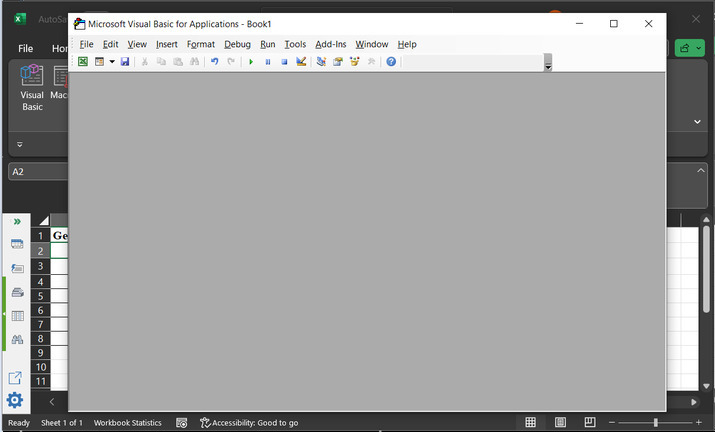### Step 4

Go to the “Insert” tab option, and then click on “Module”. This will eventually open the code area.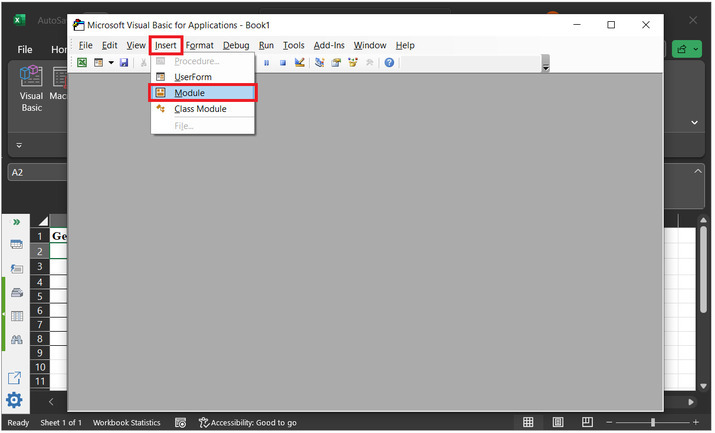### Step 5

In the opened code editor copy the below given code −

' method to calculate the unique random data
Sub UniqueRandomList ()
'  Declaring required variables
Dim nums(1 To 50) As Boolean
Dim randNums(1 To 10) As Integer
' Change 10 to the number of random numbers user wants to generate
Dim i As Integer
Dim j As Integer
' Seed the random number generator
Randomize
' for each loop expression
For i = 2 To 50
' Initialize the array to False
nums(i) = False
' Next expression
Next i
' Generate a list of unique random numbers
For i = 2 To 10 ' Change 10 to the number of random numbers you want to generate
' do loop
Do
' random numbers
randNums(i) = Int(50 * Rnd + 1)
' loop for while
Loop While nums(randNums(i)) = True
' Mark the number as used
nums(randNums(i)) = True
Next i
' Print the list of unique random numbers on a worksheet
For j = 2 To 10
' Change 10 to the number of random numbers you want to generate
Range("A" & j).Value = randNums(j)
Next j
End Sub


Please use proper code indentation to generate results without any errors. Snapshot for required code −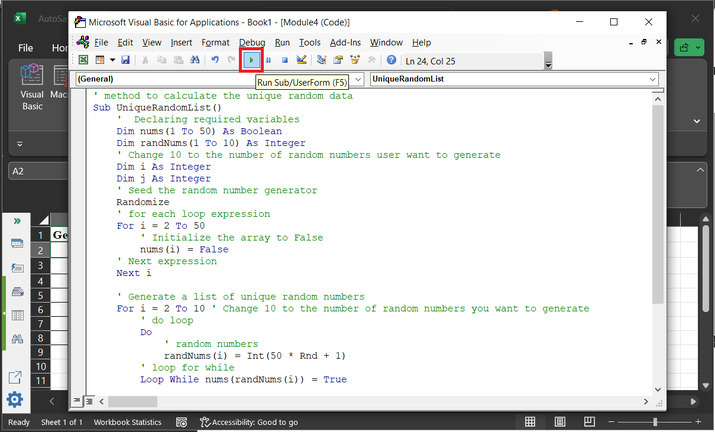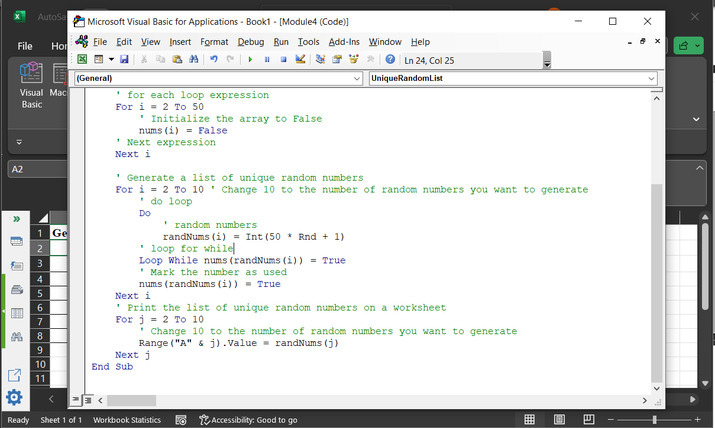### Step 6

Go back to the selected Excel sheet area, and check a list of Excel unique values that will be generated there automatically. Consider the below-depicted image for proper reference −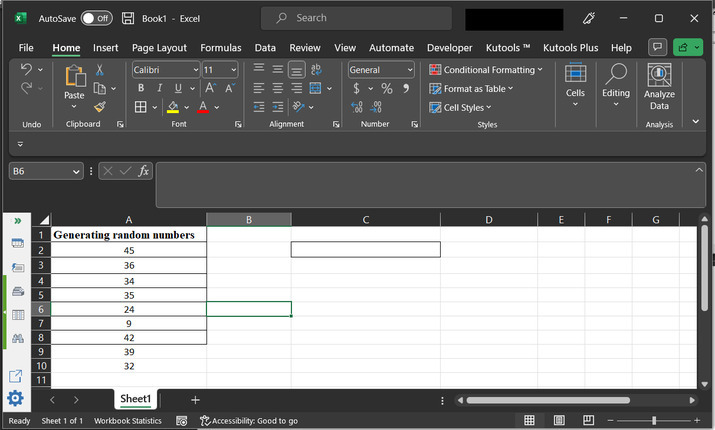## Example 2: To generate random numbers in Excel without duplicates by using the kutool

### Step 1

Again consider the same Excel sheet. Go to the “Kutools” tab → ”Editing ” under the “Editing” option, and choose “Insert”. After that select the “Insert Random Data” dialog box in Excel.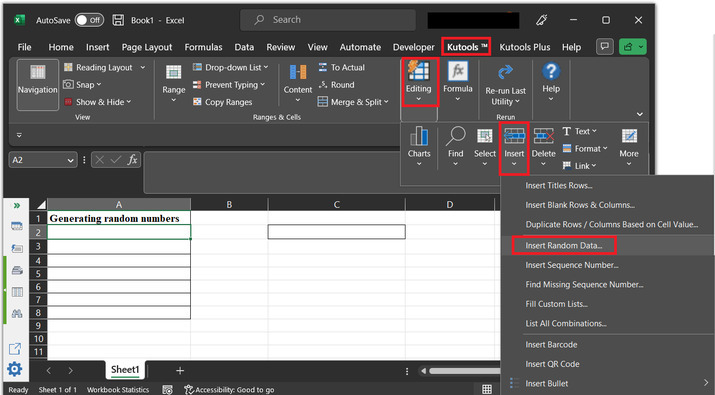### Step 2

In the “Insert Random Data” dialog box. Select the “Integer” section. In the “Integer” section, under the “From” label set the initial range value. For this example, let’s set the value to 1. After that in the “To” label enter the last data value. For this example, the considered value is 10. Select the tick to generate unique values only, and finally click on “Ok”.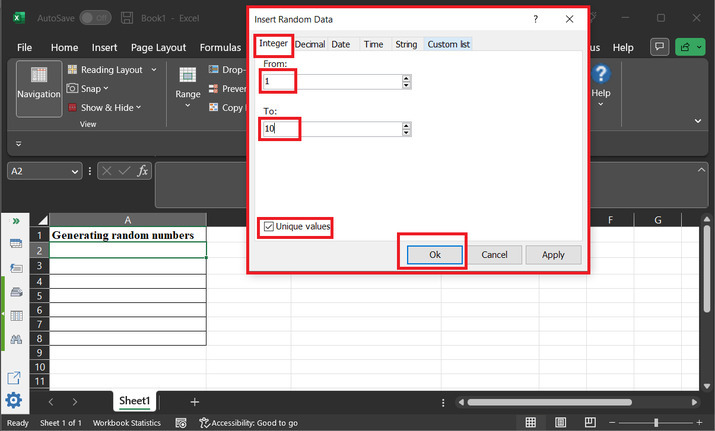### Step 3

This will display the set of required random numbers in the Excel sheet. Consider below the given Excel sheet for random values.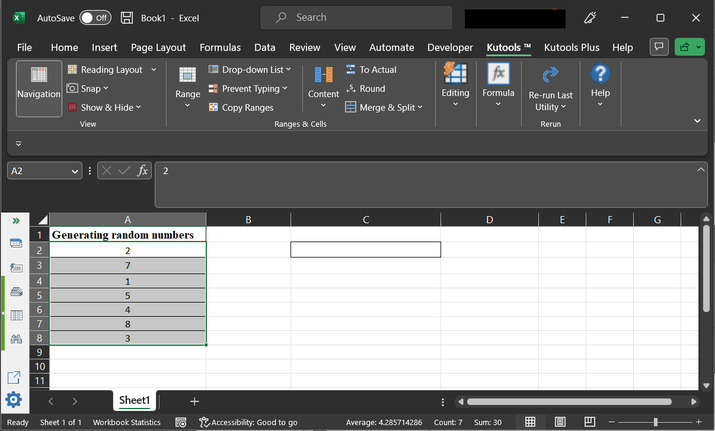## Conclusion

This article allows the users to understand the process to generate unique random numbers. The first method involves the use of VBA code, while the second method focuses on using the “Kutool” to generate the unique random values from the provided data range.

Updated on: 08-May-2023

162 Views